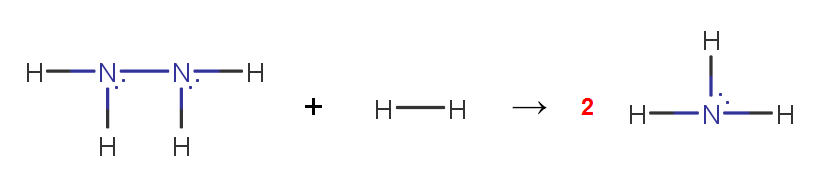# Problem: Consider the reaction between hydrazine and hydrogen to produce ammonia, N2H4(g) + H2(g) → 2 NH3(g). Use enthalpies of formation and bond enthalpies to estimate the enthalpy of the nitrogen-nitrogen bond in N2H4.

###### FREE Expert Solution

To calculate the ΔH°rxn using bond energies, we’re going to use the following equation:

N2H4(g) + H2(g) → 2 NH3(g)90% (85 ratings)###### Problem Details

Consider the reaction between hydrazine and hydrogen to produce ammonia, N2H4(g) + H2(g) → 2 NH3(g). Use enthalpies of formation and bond enthalpies to estimate the enthalpy of the nitrogen-nitrogen bond in N2H4.

Frequently Asked Questions

What scientific concept do you need to know in order to solve this problem?

Our tutors have indicated that to solve this problem you will need to apply the Bond Energy concept. You can view video lessons to learn Bond Energy. Or if you need more Bond Energy practice, you can also practice Bond Energy practice problems.

What professor is this problem relevant for?

Based on our data, we think this problem is relevant for Professor Curtis' class at UNC.